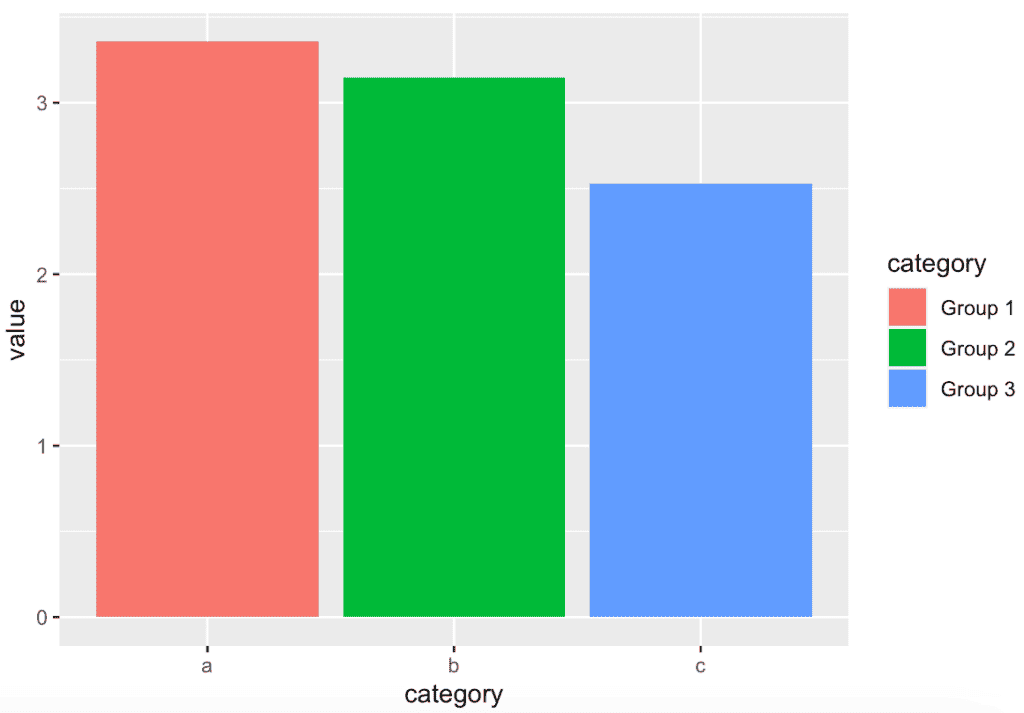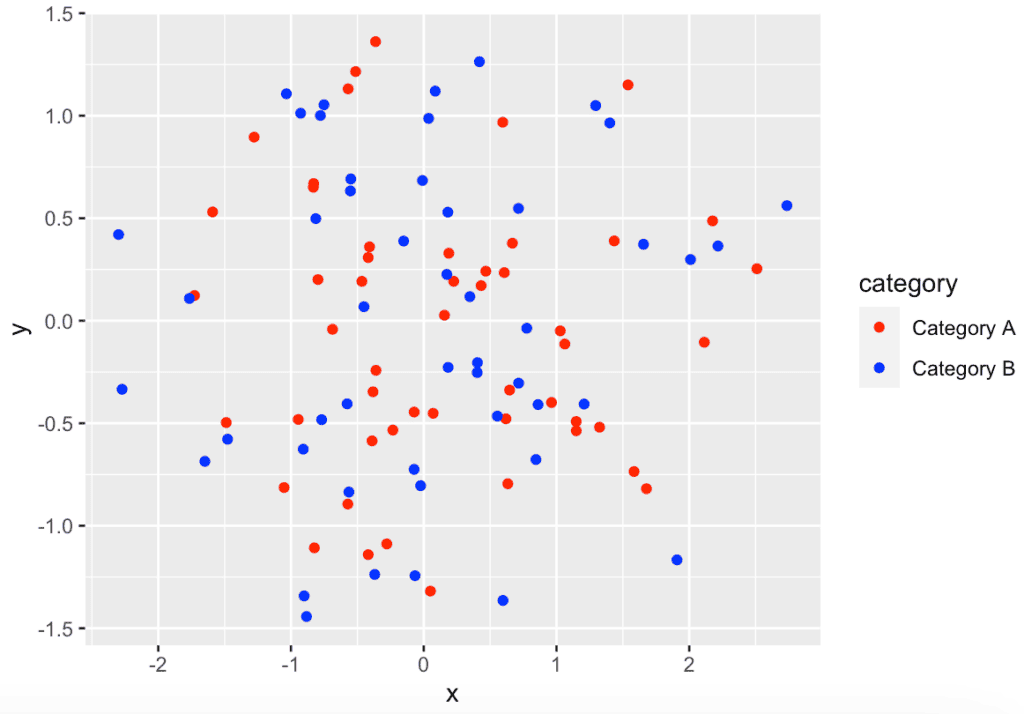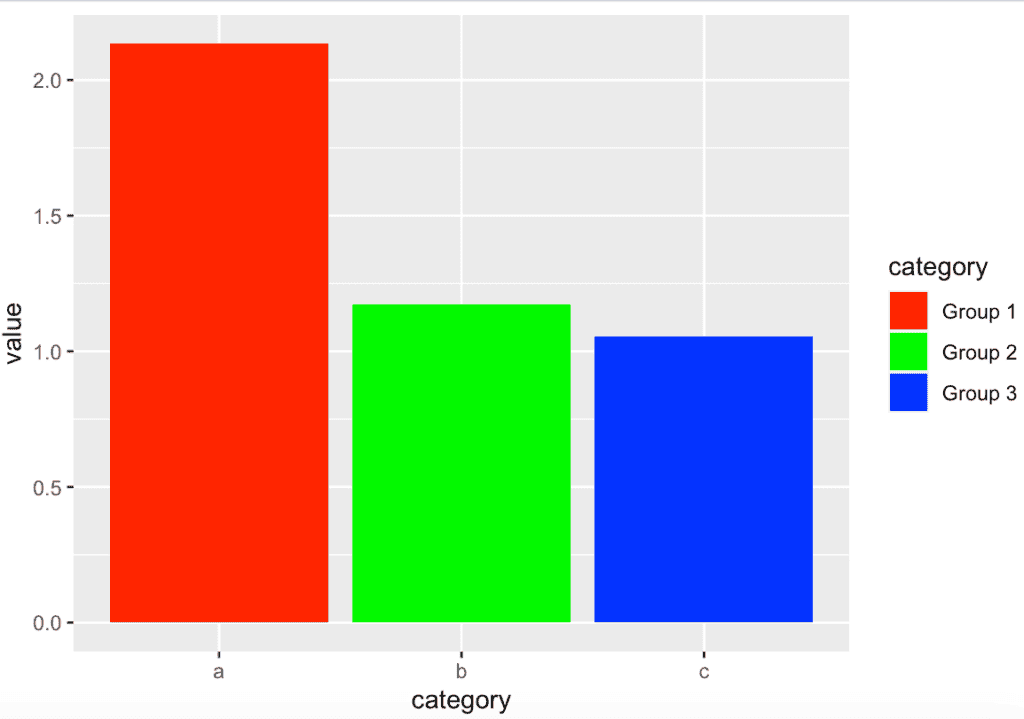# How to Change Legend Labels in ggplot2 (With Examples)

The scale_fill_discrete() function is “used to change the legend labels for discrete fill aesthetics in ggplot2.” You can use the labels argument to specify the new labels.

## Syntax

``p + scale_fill_discrete(labels=c('label1', 'label2', 'label3', ...))``

## Example 1: Using scale_fill_discrete() function

``````# Load the ggplot2 library
library(ggplot2)

# Create a sample data frame
df <- data.frame(
category = rep(letters[1:3], each = 4),
value = runif(12)
)

# Create a bar plot
p <- ggplot(df, aes(x = category, y = value, fill = category)) +
geom_bar(stat = "identity")

# Customize the fill legend labels using scale_fill_discrete()
plot <- p + scale_fill_discrete(labels = c("Group 1", "Group 2", "Group 3"))

# Display the plot
plot``````

OutputIn this example, we’re creating a bar plot with three categories and then using scale_fill_discrete() to change the legend labels for the fill aesthetic to “Group 1,” “Group 2,” and “Group 3.”

## Example 2: Changing Color Legend Labels

``````library(ggplot2)

# Create sample data
df <- data.frame(x = rnorm(100), y = rnorm(100),
category = sample(letters[1:2], 100, replace = TRUE))

# Plot with custom color legend labels
plot1 <- ggplot(df, aes(x = x, y = y, color = category)) +
geom_point() +
scale_color_manual(values = c("red", "blue"), labels = c("Category A", "Category B"))

# Display the plot
plot1``````

Output## Example 3: Changing Fill Legend Labels in a Bar Plot

``````# Create sample data
df <- data.frame(category = rep(letters[1:3], each = 4), value = runif(12))

# Bar plot with custom fill legend labels
plot2 <- ggplot(df, aes(x = category, y = value, fill = category)) +
geom_bar(stat = "identity") +
scale_fill_manual(values = c("red", "green", "blue"),
labels = c("Group 1", "Group 2", "Group 3"))

# Display the plot
plot2``````

OutputThat’s it!

## Related posts

How to Change the Legend Title in ggplot2

How to Change Legend Size in ggplot2

How to Remove a Legend in ggplot2